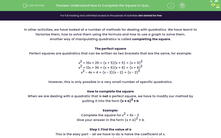# Understand How to Complete the Square in Quadratics

In this worksheet, students will practise completing the square for quadratic expressions.Key stage:  KS 4

Year:  GCSE

GCSE Subjects:   Maths

GCSE Boards:   AQA, Eduqas, Pearson Edexcel,

Curriculum topic:   Algebra

Curriculum subtopic:   Notation, Vocabulary and Manipulation

Popular topics:   Algebra worksheets

Difficulty level:#### Worksheet Overview

In other activities, we have looked at a number of methods for dealing with quadratics. We have learnt to factorise them, how to solve them using the formula and how to use a graph to solve them.

Another way of manipulating quadratics is called completing the square.

The perfect square

Perfect squares are quadratics that can be written as two brackets that are the same, for example:

x2 + 10x + 25 = (x + 5)(x + 5) = (x + 5)2

x2 + 12x + 36 = (x + 6)(x + 6) = (x + 6)2

x2 - 4x + 4 = (x - 2)(x - 2) = (x - 2)2

However, this is only possible in a very small number of specific quadratics.

How to complete the square

When we are dealing with a quadratic that is not a perfect square, we have to modify our method by putting it into the form (x ± a)2 ± b

Example:

Complete the square for x2 + 6x - 2

Step 1: Find the value of a

This is the easy part - all we have to do is halve the coefficient of x.

For our question, we have +6x, this means that a is 3.

(x + 3)2 ± b

Step 2: Find the value of b

This takes a bit more thought.

If we look at the expression we have, you will notice that we have a perfect square at the start which we can expand:

(x + 3)2 = (x + 3)(x + 3) = x2 + 6x + 9

You will notice that the only difference between this perfect square and our original question is that the last number is -2.

The question we need to ask is how do we get from the 9 we have, to the -2 we want.

We have to subtract 11.

This means that b is -11.

We can now write our quadratic as:

x2 + 6x – 2 = (x + 3)2 - 11

Let's have a go at some questions now.

### What is EdPlace?

We're your National Curriculum aligned online education content provider helping each child succeed in English, maths and science from year 1 to GCSE. With an EdPlace account you’ll be able to track and measure progress, helping each child achieve their best. We build confidence and attainment by personalising each child’s learning at a level that suits them.

Get started